#There are 366 different Starters of The Day, many to choose from. You will find below some starters on the topic of Mixed. A lesson starter does not have to be on the same topic as the main part of the lesson or the topic of the previous lesson. It is often very useful to revise or explore other concepts by using a starter based on a totally different area of Mathematics.

Main Page

### Mixed Starters:A game based around the concept of factors and abundant numbers.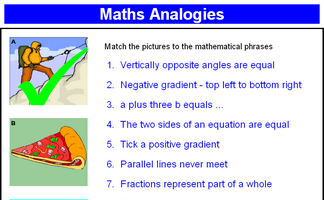Make more of analogies to help remember mathematical concepts.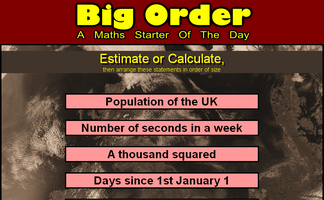Estimate or calculate then put the large numbers in order of size.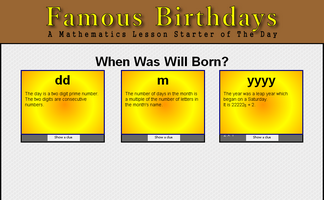Work out the date Will was born by answering some number questions.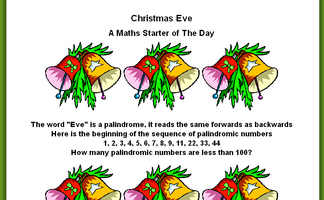Is there a pattern in the number of palindromic numbers to be found less than powers of 10?Can you use the digits on the left of this clock along with any mathematical operations to equal the digits on the right?Questions about the Small LEDs used to make up the digits on a calculator display.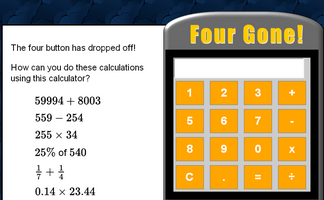An activity involving a broken calculator which is missing the four button. Can you evaluate the given expressions without using the four?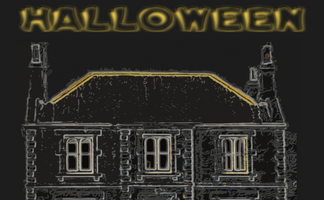Five problems with a Halloween theme.Everyone in the class holds up an item to show which is the correct answer to the multiple choice question.Loosely based on the famous television show, how many questions cn you come up with for a given answer?Four mathematical questions to think about at the beginning of the lesson.The 31st of December is the last day of the year. What mathematical lasts do you know?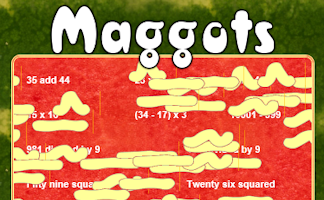Do as many of the calculations as possible before the maggots infest!A small crossword puzzle containing mathematical words.The starter is a PowerPoint file containing a twenty question mental arithmetic test. It will advance from one question to the next automatically.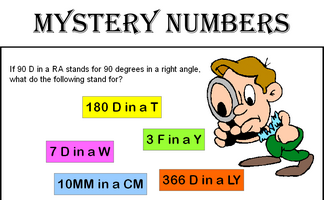Can you recognise the mystery numbers from the clues?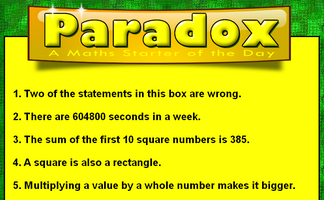Can you solve this paradox by agreeing whether the statements are right or wrong?Stand at the point between the classroom walls to represent a given number.It is called Refreshing Revision because every time you refresh the page you get different revision questions.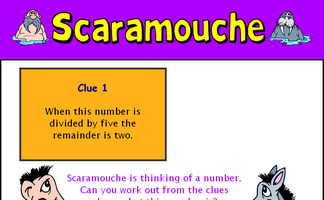Can you work out from the five clues given what the mystery number is?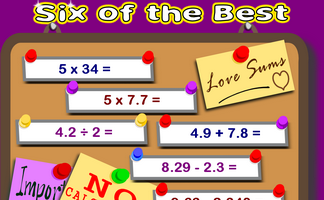Six calculations to perform without a calculator.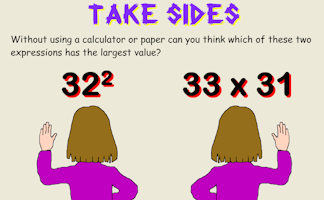Put up your right hand or left hand depending on the expressions that appears.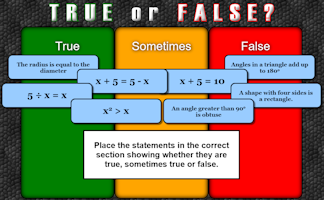An activity designed to address common misconceptions.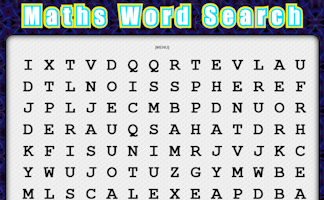Create your own mathematical word search for the whole class to enjoy.

## Exercises#### Algebraic Perimeters

Questions about the perimeters and areas of polygons given as algebraic expressions.#### Back To School

A list of suggested activities and resources for the first few lessons of the new school year.#### Beat The Clock

It is a race against the clock to answer 30 mental arithmetic questions. There are nine levels to choose from.#### Chain Challenge

How fast can you perform all of the given operations without using a calculator?#### Emergency Maths Lessons

Here are some mathematics lesson plans to be used on the rare occasions when a class is left without their normal teacher and you are stepping in at a moment's notice.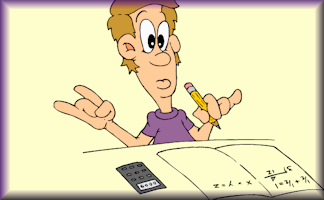#### Exam Tips

Some advice, tips and tricks for a Maths exam presented in the form of a poem for a teacher to share with the class.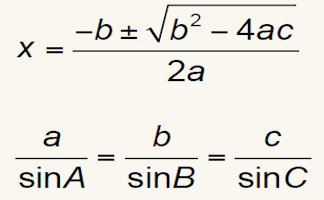#### Formulae to Remember

The traditional pairs or pelmanism game adapted to test recognition for formulae required to be memorised for GCSE exams.#### IB AA SL Formulae Pairs

Find the matching pairs of formulae and their names in this game designed for IB AA SL students during their final revision.#### Kim's Quiz

The traditional memory game played with mathematical statements.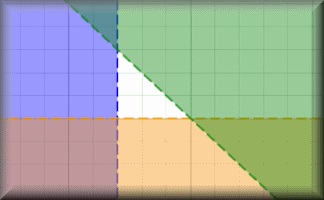#### Linear Programming

A selection of linear programming questions with an interactive graph plotting tool.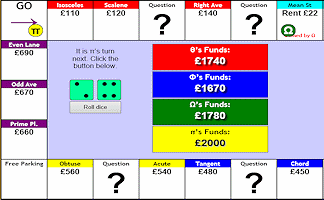#### Mathopoly

A game of buying and selling property with maths questions thrown in for good measure.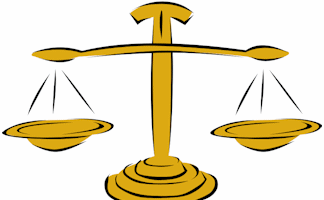#### Maths Analogies

Make more of analogies to help remember mathematical concepts.#### Maths Bingo

This application will pick the bingo numbers for you and present each of the numbers as a mathematical expression.#### Maths General Knowledge Quiz

Questions about general mathematical facts and concepts.#### Maze

Each visitor to this page has a unique maze constructed for them to find their way through answering mathematical questions on the way.#### Mini Whiteboard

If each pupil has an iPad, laptop or slate this provides an alternative to 'hands up'.#### Mystery Numbers

If '7 D in a W' stands for 7 days in a week, what do you think these mystery numbers are?#### Numbasics

A daily workout strengthening your ability to do the basic mathematical operations efficiently.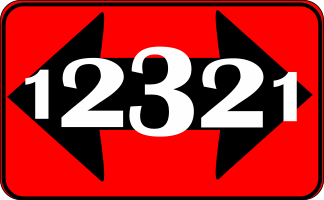#### Number Palindromes

A collection of activities based around the theme of palindromic numbers.#### Number Skills Inventory

A checklist of basic numeracy techniques that every pupil should know.#### Numskull

Interactive, randomly-generated, number-based logic puzzle designed to develop numeracy skills.#### Pentransum

Mathematical questions with five possible answers. If you get 20 correct you can add your own question to the database.#### Playing Card Maths

Imagine you are on a desert island with nothing but a pack of playing cards. Do you have to stop learning mathematics?#### Quickulations

A mental arithmetic visual aid that displays random calculations then after a few seconds displays the answers.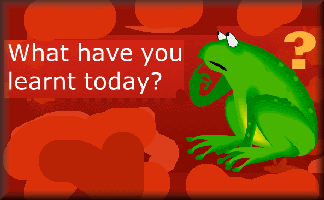#### Random Recap

Randomly chosen questions to project onto the whiteboard for the end of the lesson plenary.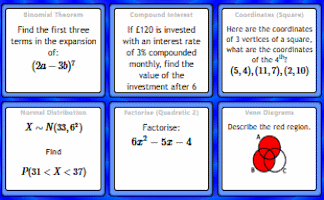#### Refreshing Revision

Every time you refresh the page you get different revision questions for whole class retrieval practice.#### Snappy Sorting

Sort the numbers in to the correct order as fast as you can.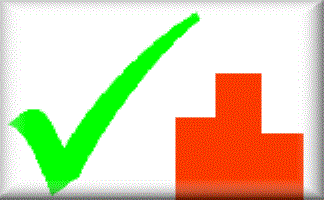#### Student Voting

This system allows students to vote or choose a response to a question posed by a teacher.#### Timer

A simple visual aid which acts as a stopwatch recording the number of minutes and seconds that have passed or are left.#### Today's Date

A customisable lesson Starter featuring today's date.#### Topic Test

Ten multiple choice questions randomly chosen from those suggested by people from all around the world.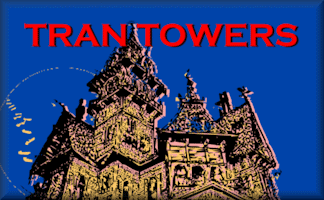#### Tran Towers

An adventure game requiring students to solve puzzles as they move through the old mansion.#### Tran Tunnels

A mini adventure game containing maths puzzles and problems. Find your way through the maze of tunnels to find Goldberg's magic harpsicord.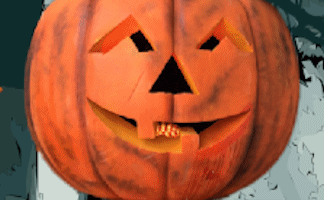#### Trick Or Treat

A mathematical true or false general knowledge activity for Halloween.#### True or False?

Arrange the given statements in groups to show whether they are always true, sometimes true or false.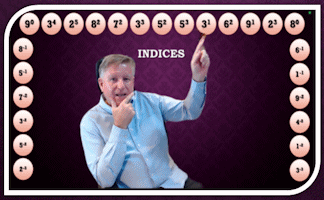#### Video Backgrounds

A selection of images which might be useful as the background of your remote Maths teaching video stream.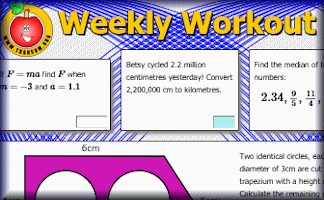#### Weekly Workout

GCSE-style questions for regular practice designed for students targeting grades 3 to 5 (C/D) of the GCSE Mathematics examination.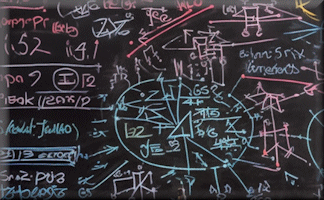#### Where am I with Algebra?

Find out how developed your algebra skills are and then take them to the next level.#### Yohaku Puzzles

Fill in the blank spaces so that the cells give the sum or the product shown in each row and column.

### Search

The activity you are looking for may have been classified in a different way from the way you were expecting. You can search the whole of Transum Maths by using the box below.

Have today's Starter of the Day as your default homepage. Copy the URL below then select
Tools > Internet Options (Internet Explorer) then paste the URL into the homepage field.

Set as your homepage (if you are using Internet Explorer)

Do you have any comments? It is always useful to receive feedback and helps make this free resource even more useful for those learning Mathematics anywhere in the world. Click here to enter your comments.For All: